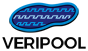### ProfileHome News Activity About/Contact Major Tools Dinotrace Verilator Verilog-mode Verilog-Perl Other Tools IPC::Locker Parallel::Forker Voneline General Info Papers

## Issue #433

### indenting for some forms of SystemVerilog constraints is wrong/odd

Status:
Closed
Priority:
Normal
Assignee:
Category:
Indents
% Done:

90%

Estimated time:
2.00 h

Description

indentation for several different forms of SV constraints is wrong or odd; specifically, empty constraints are odd, as are constraints with "if" followed by braces

It acts like it doesn't understand the braces (i.e. {})

Here's an example:

class data; rand integer data1; rand integer data2,data3; rand reg [31:0] foo;

``````constraint basic_c {
endclass // data``````
``````//empty constraint
}``````
``````constraint complex_c {
data1 <= 100;
foo inside {[40:999]};
if(foo < 87)
data2  10;
if(data2  76) {
data3  8;
}
}``````
``````constraint implication_c {
data1  10 -> data3 >= -1;
}``````
``````function new();
data1 = 0;
data2 = 78;
endfunction // new``````

### History

wow. the html (or php?) code "displayer" really mangled that code. That's not at all what I pasted.

I'll try and with a pre block...

```class data;
rand integer data1;
rand integer data2,data3;
rand reg [31:0] foo;

constraint basic_c {

//empty constraint
}

constraint complex_c {
data1 <= 100;
foo inside {[40:999]};
if(foo < 87)
data2 == 10;
if(data2 == 76) {
data3 == 8;
}
}

constraint implication_c {
data1 == 10 -> data3 >= -1;
}

function new();
data1 = 0;
data2 = 78;
endfunction // new
endclass // data
```

#### #2 Updated by Brad Parkeralmost 8 years ago

I made a small set of changes which solve "my problem" (as in, "works for me!" :-) with systemverilog constraints. I now get indenting which looks rational...

I'm not 100% happy with the changes, but they are at least minimal and clean. These diffs are against 735

```--- verilog-mode.el.orig        2012-04-02 11:28:24.530560000 -0700
+++ verilog-mode.el     2012-04-02 13:50:26.922656000 -0700
@@ -5315,7 +5315,13 @@
((eq type 'defun)
(list type 0))
(t
-          (list type (verilog-current-indent-level))))))))
+          ;;(message "block point %s starting_position %s indent %s inside-constraint %s"
+          ;;       (point) starting_position
+          ;;       (verilog-current-indent-level-pos starting_position)
+          ;;       (verilog-inside-constraint-pos-p starting_position))
+          (if (verilog-inside-constraint-pos-p starting_position)
+              (list type (verilog-current-indent-level-pos starting_position))
+            (list type (verilog-current-indent-level)))))))))

(defun verilog-wai ()
"Show matching nesting block for debugging."
@@ -5921,6 +5927,7 @@
(verilog-at-constraint-p)
)
nil)))
+
(defun verilog-at-close-constraint-p ()
"If at the } that closes a constraint or covergroup, return true."
(if (and
@@ -5929,7 +5936,8 @@

(save-excursion
(verilog-backward-ws&directives)
-       (if (equal (char-before) ?\;)
+;;     (if (equal (char-before) ?\;)
+       (if (or (equal (char-before) ?\;) (equal (char-before) ?\}))
(point)
nil))))

@@ -5941,12 +5949,26 @@
(forward-list)
(progn (backward-char 1)
(verilog-backward-ws&directives)
-               (equal (char-before) ?\;))))
+;;             (equal (char-before) ?\;))))
+               (or (equal (char-before) ?\;) (equal (char-before) ?\})))))
;; maybe
(verilog-re-search-backward "\\<constraint\\|coverpoint\\|cross\\>" nil 'move)
;; not
nil))

+(defun verilog-inside-constraint-pos-p (pos)
+  "If inside a constraint or coverpoint definition, return true, moving point to constraint."
+  (save-excursion
+    (let (par-pos)
+      (beginning-of-line)
+      (setq par-pos (verilog-parenthesis-depth))
+      (while par-pos
+       (goto-char par-pos)
+       (beginning-of-line)
+       (setq par-pos (verilog-parenthesis-depth)))
+      (skip-chars-forward " \t")
+      (verilog-re-search-forward "\\<constraint\\>" pos 'move))))
+
(defun verilog-at-struct-p ()
"If at the { of a struct, return true, moving point to struct."
(save-excursion
@@ -6227,6 +6249,21 @@
indent-str                         ; Return indent data
))

+(defun verilog-current-indent-level-pos (pos)
+  "Return the indent-level of the current statement."
+  (save-excursion
+    (let (par-pos)
+      (goto-char pos)
+      (beginning-of-line)
+      (setq par-pos (verilog-parenthesis-depth))
+      (if par-pos
+         (progn
+           (goto-char par-pos)
+           (beginning-of-line)
+           (skip-chars-forward " \t")
+           (current-column))
+       0))))
+
(defun verilog-current-indent-level ()
"Return the indent-level of the current statement."
(save-excursion

```

#### #3 Updated by Wilson Snyderalmost 8 years ago

As you said, this seems better, but is not perfect. Even so I was ready to commit it, however it breaks basic Verilog indentation with the following, so unfortunately I can't merge it. Attached is a modified version of your patch that just changes a few naming and related issues if you'd like to fix that one instead.

```  always_comb
begin
case (in1)
4'b0001 :     begin
out1  = in2;
end
default       :       begin
out1  = {4{1'b0}};
end  // This mis-indents
endcase
end
```

#### #4 Updated by Brad Parkeralmost 8 years ago

I noticed that also (but only yesterday). I'll fix that and resubmit. thanks!

#### #5 Updated by Austin Harrisabout 7 years ago

Can you please assign someone to fix this? Any OVM/UVM verification code is extremely annoying to deal with because of this.

#### #6 Updated by Wilson Snyderabout 7 years ago

The way things get fixed with open source is you "assign" yourself to fix it.

Bruce <> submitted another patch (attached), but this still breaks basic indents so cannot be merged. Perhaps you could ask if he needs assistance getting this ready.

#### #7 Updated by Austin Harrisover 6 years ago

Hello, the constraints indenting is working great now in most cases, however I am seeing an issue with the following snippet:

```if (x)
`ovm_do_with(my,
{ y == 1;
z == 2;
});
```

Could anyone give me any guidance on where to look to go about fixing this?

#### #8 Updated by Alex Reedalmost 5 years ago

• Category set to Indents
• Assignee set to Wilson Snyder
• % Done changed from 0 to 90
• Estimated time set to 2.00 h

Wilson, Please merge https://github.com/acr4/verilog-mode/commits/bugfix-433 which fixes the supplied test-case. emacs and xemacs regressions pass (for me anyway...). Thanks, -Alex

#### #9 Updated by Wilson Snyderalmost 5 years ago

• Status changed from New to Closed
• Assignee changed from Wilson Snyder to Alex Reed

Thanks again, pushed to git.

Also available in: Atom